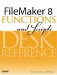# Combination()

Category: Number

 Syntax: Combination ( setSize; numberOfChoices )

Parameters:

setSizeNon-negative numeric value (or an expression that results in one).

numberOfChoicesNon-negative numeric value (or an expression that results in one).

Data type returned: Number

Description:

Returns the number of ways to uniquely choose numberOfChoices items from a set of size setSize.

The formula used to determine the Combination value is n! / (n-x)! * x!, where n = set size, x = number of choices.

The numbers returned by the Combination function are the coefficients of the binomial expansion series. Useful in statistics, combinatorics, and polynomial expansions, the values returned by this function are referred to as combination coefficients. They form Pascal's triangle.

``` (x + y)4 = 1x4 + 4x3y + 6x2y2 + 4xy3 + 1y4
Combination ( 4; 0 ) = 1
Combination ( 4; 1 ) = 4
Combination ( 4; 2 ) = 6
Combination ( 4; 3 ) = 4
Combination ( 4; 4 ) = 1
```

Examples:

Function

Results

Combination ( 4; 2 )

Returns 6 because there are six ways of selecting two items from a set of four items. Given set {A, B, C, D}, these subsets would be {AB, AC, AD, BC, BD, CD}.

Combination ( x; 0 )

Returns 1 for any x representing the empty set.

Combination ( x; x )

Returns 1 for any x.

( 13 * 12 * Combination ( 4; 2 ) * Combination ( 4; 3 )) / Combination ( 52; 5 )

Returns 0.00144057..., which is the probability of being dealt a full house in five-card poker (less than a 1% chance).FileMaker 8 Functions and Scripts Desk Reference
ISBN: 0789735113
EAN: 2147483647
Year: 2004
Pages: 352